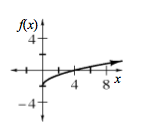### Home > CCA2 > Chapter 1 > Lesson 1.2.2 > Problem1-86

1-86.

Make a complete graph of the function $f(x)=\sqrt{x}-2$ , label its $x$- and $y$-intercepts, and describe its domain and range

Make a table.

What is the smallest value of $x$ you can use?

Try using $0$, $1$, $2$, $3$, $4$, and $9$ as $x$-values. What happens when you try $−1$ or $−4$?

What is the smallest output value possible?